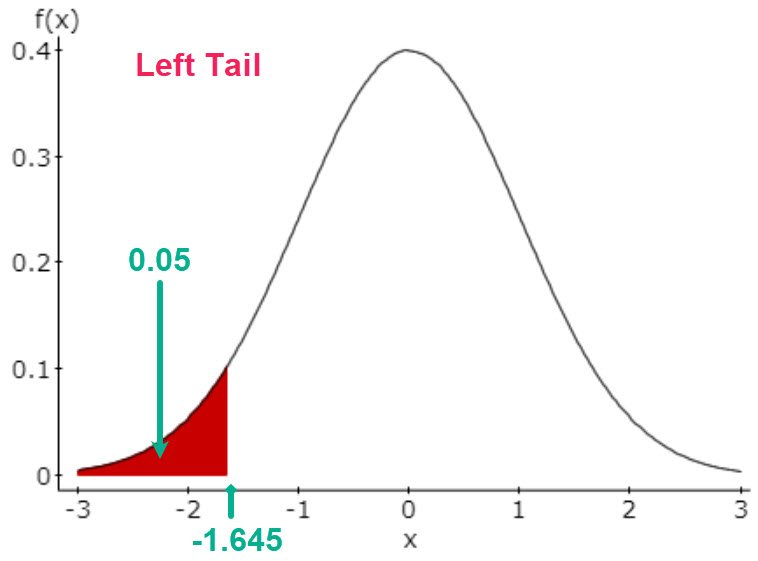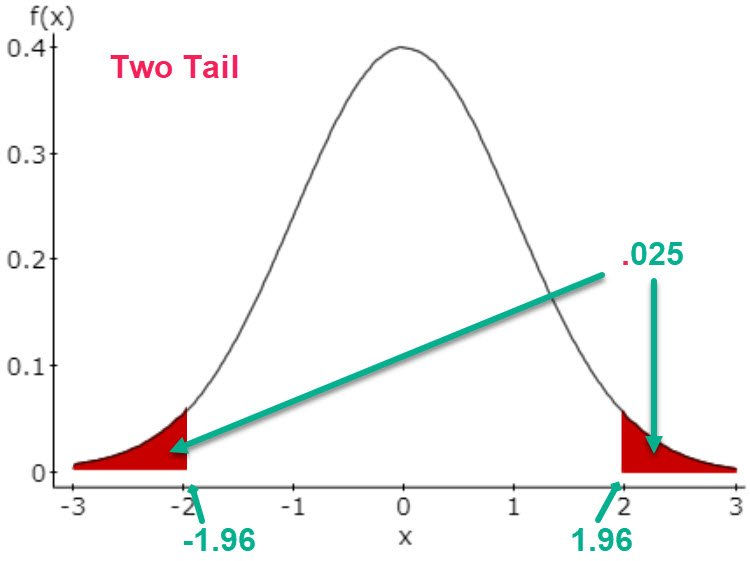# Setting up Hypothesis Tests – Three Useful Tables

Step 1: Words to Symbols Table

Read the problem carefully, looking for key phrases and words which will identify the claim. Look for those key phrases in the table below to determine if the claim is the null or alternative hypotheses. It can be either. Because the null and alternative must be complements, finding one will also give you the other as they will be in the same row.

For an example, one problem includes this sentence: “The test results prompts a state school administrator to declare that the mean score for the state’s 8th graders is more than 260.” The “more than” phrase is a key to recognizing the claim is “more than” which is in the Alternative Hypothesis column in Row 3. The Math Operator in that cell is >. Thus, the claim is: µ > 260. The math operator in the claim always indicates the tail of the test, e.g. a > symbol points to the right, so this is a right tail test.

In the center column on that row, row 3, you see the two hypotheses statements written in mathematical format. You just need to substitute the claimed value for x to complete them: H0: μ 260, Ha: μ > 260.Tail of the Test Table

The tail of the hypothesis test is determined by looking at the math operator in the alternative hypothesis. I like to think of the direction in which the math operator is pointing. A less than operator, <, seems to be pointing to the left in my mind. Thus, it indicates a left tail test. A greater than symbol, >, points right and indicates a right tail test. The not equal, ≠, does not point either way, so that clues me to think of a two-tail test.The following images show a left tail, two tail, and right tail sketches for a significance level, alpha, of 0.05.

For a left or right tail test, we put all of alpha in that tail, but in the two-tail test, we put half of alpha, or 0.025 in this example, in each tail. Using those probabilities, we find the critical values.The critical z-value in the left tail test is -1.645 and any z test statistic that is smaller or to the left of that value falls in the rejection area. Similarly, any z test statistic greater than +1.645 falls to the right of the critical z-value and is in that rejection area.For the two-tail test, the test statistic must have a larger absolute value to fall in one of the rejection areas. Here, the z test statistic must be less than -1.96 or greater than + 1.96.The logic of why the test statistic must have a larger absolute value is that we set the level of significance, alpha, by deciding how confident we want to be in the decision we make. Here, we want to be 95% sure we make the right decision or just have a 5% chance of making a wrong decision, a Type 1 error of rejecting a true Null hypothesis. Because the total area/probability under the normal distribution must be 1, the area between the two tails must be 0.95 if the total chance of being wrong is alpha or 5%. That means that half of alpha must go into each tail.

Although the images above are for a z-test, similar sketches could be made for a t or a chi-square test.

How to State the Conclusion

After you have completed the statistical analysis and decided to reject or fail to reject the Null hypothesis, you need to state your conclusion about the claim. To get the correct wording, you need to recall which hypothesis was the claim. If the claim was the null, then your conclusion is about whether there was sufficient evidence to reject the claim. Remember, we can never prove the null to be true, but failing to reject it is the next best thing.

If the claim is the alternative hypothesis, your conclusion can be whether there was sufficient evidence to support (prove) the alternative is true.